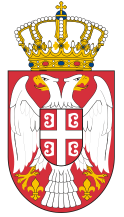Dokument se učitava

Engineering MathematicsCourse code: 100307 | 6 ECTS credits

Basic information
Level of Studies: Undergraduate applied studies
Year of Study: 1
Semester: 1
Requirements:
Goal: Acquiring the necessary mathematical knowledge for professional subjects in the field of electrical engineering, expanding mathematical education, developing creative thinking and creating a basis for understanding quantitative relationships among phenomena.
Outcome: Students will be able to solve complex mathematical problems related to the application of modern mathematical methods in the field of electrical engineering.
Contents of the course
Theoretical instruction:
1. Complex numbers.
2. Determinants, characteristics, and calculation.
3. The notion of matrix, properties, and operations. Inverse matrix. Matrix rank.
4. Methods for solving the system of linear equations.
5. Domain of function, zeros and sign of function, y-axis cross-section, parity and periodicity.
6. 1st colloquium.
7. Concept and calculation of the limit value of a function. Function asymptotes (vertical, horizontal, slant).
8. Derivatives and differentials of function. Flatness and convexity of function.
9. Basic Derivative Theorems: L'Hospital Rule, Taylor and MacLauren polynomial. Geometric interpretation of the derivative.
10. Testing the flow and sketching graphics of the function.
11. Undetermined and determined integrals - basic properties and calculation.
12. Integral of rational functions and integrals that are reduced to the integral of rational functions.
13. Application of a determined integral.
14. 2nd colloquium.
15. Repetition of the entire material.
Practical instruction (Problem solving sessions/Lab work/Practical training):
1. Practical instruction is followed by a lecture program. Getting acquainted with the MATLAB software package in laboratory exercises.
Textbooks and References
1. A. Savić, Z. Mišković, S. Štrbac-Savić, Matematika 1, VIŠER, Beograd, 2013.
2. A. Savić, S. Štrbac-Savić, I. Stefanović, Matematika 1 i Matlab, VIŠER, Beograd, 2015.
3. I. Kovačević, A .Savić, Inženjerska matematika, Viša elektrotehnička škola, Beograd, 2005.
Number of active classes (weekly)
Lectures: 3
Practical classes: 3
Other types of classes: 0
Grading (maximum number of points: 100)
Pre-exam obligations
Points
activities during lectures
0
activities on practial excersises
20
seminary work
0
colloquium
25
Final exam
Points
Written exam
25
Oral exam
30© 2019 School of Electrical and Computer Engineering of Applied Studies, Belgrade
Vojvode Stepe 283, office@viser.edu.rs, +381 11 2471 099